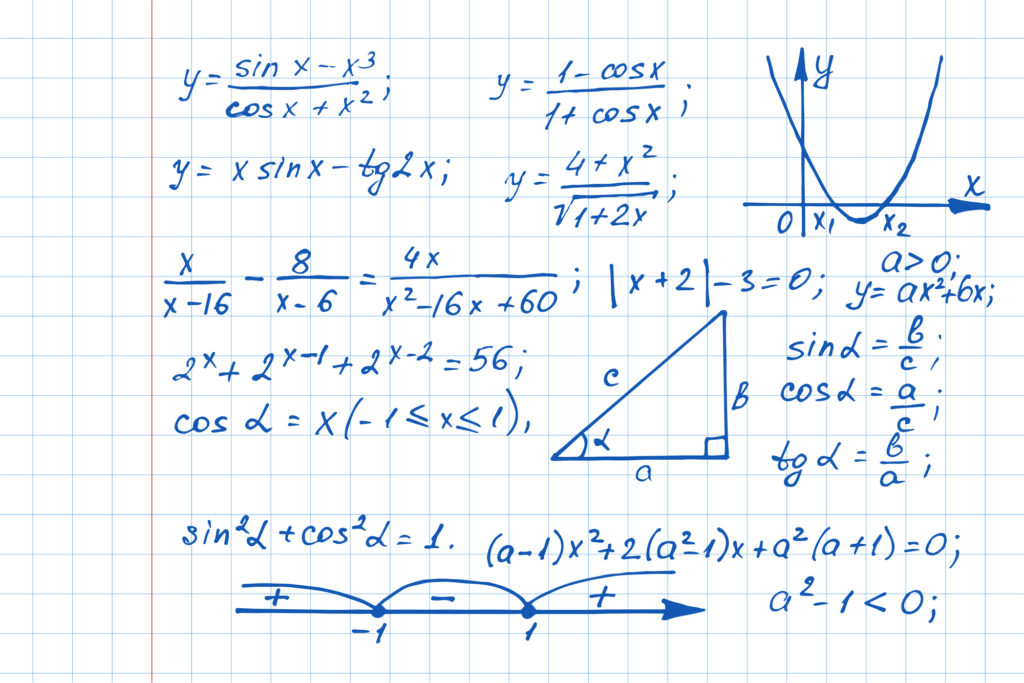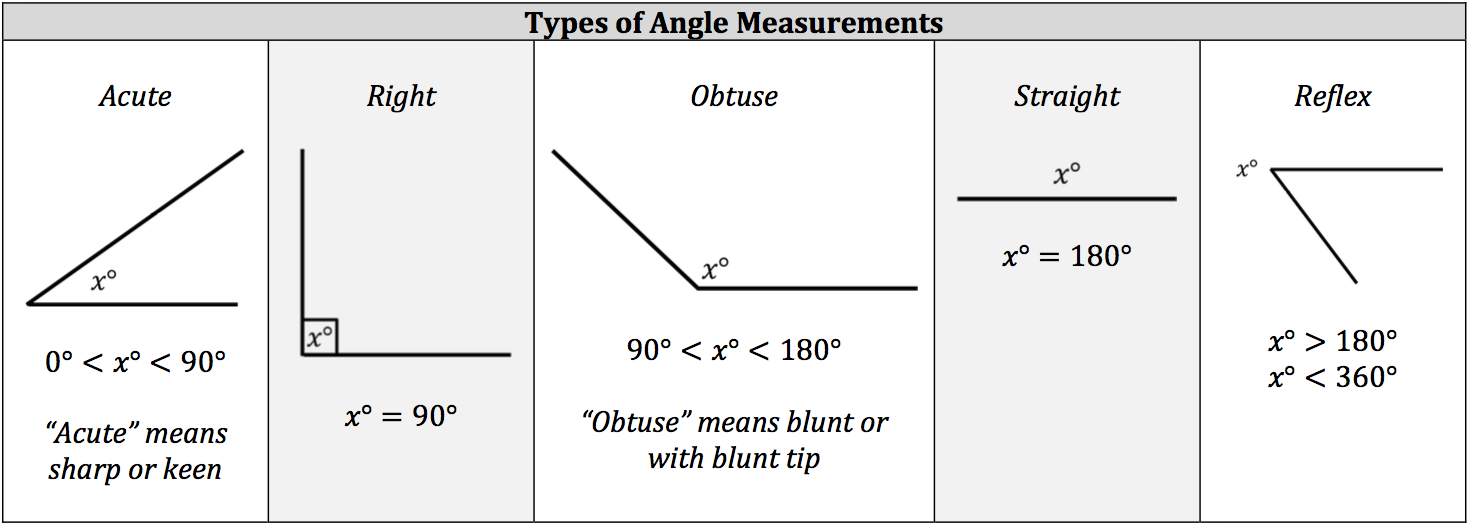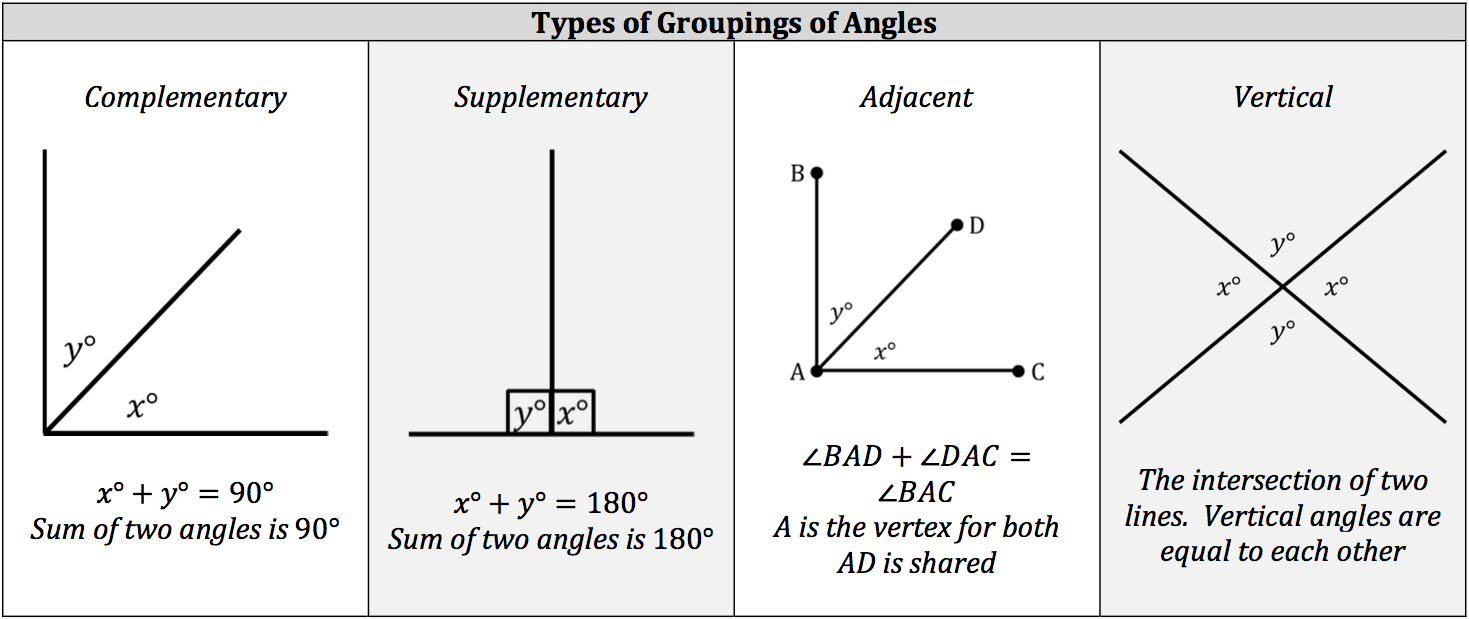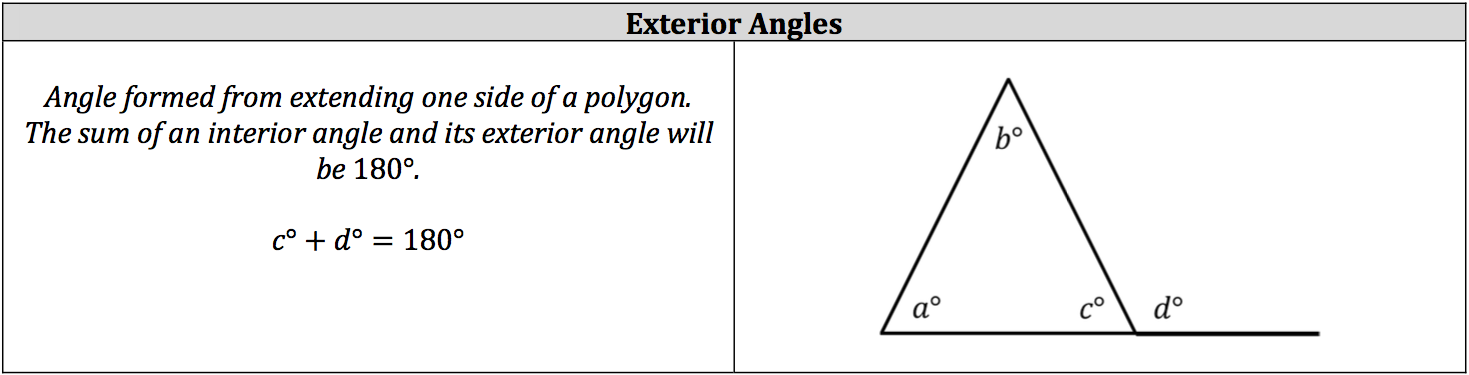An angle is formed when two rays share the same endpoint, which is called the vertex of the angle. In geometric notation, the vertex is the middle letter in the name of the angle. Each angle has a degree value, which is the measurement of the size of the angle, and the degree value determines its angle type. Groupings of angles are labeled by the sum of their angles or how they are formed.

## 5 Basic Angle Types

You should be familiar with these five basic angle types:

• Acute Angle = Less than 90°
• Right Angle = Exactly 90°
• Obtuse = Between 90° and 180°
• Straight = Exactly 180°### Interior Angle Groupings## Understanding Exterior Angles